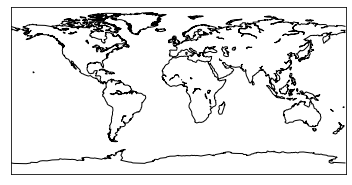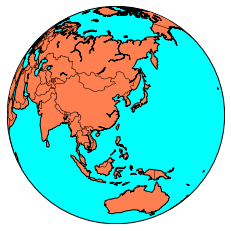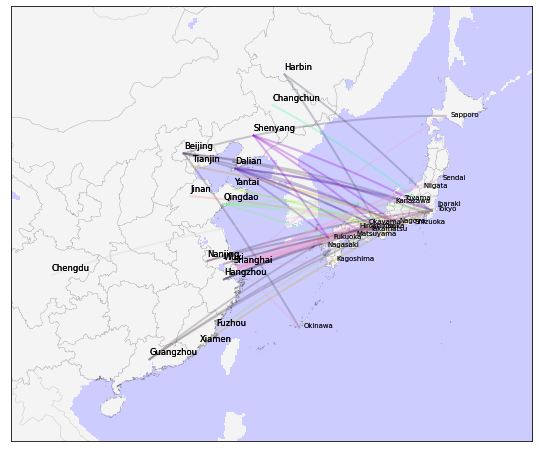## Basemap Introduction

Basemap is a toolkit under the Python visualization library Matplotlib. its main function is to draw 2D maps, which are important for visualizing spatial data. basemap itself does not do any plotting, but provides the ability to transform coordinates into one of 25 different map projections. Matplotlib can also be used to plot contours, images, vectors, lines, or points in transformed coordinates. basemap includes the GSSH coastline dataset, as well as datasets from GMT for rivers, states, and national boundaries. These datasets can be used to plot coastlines, rivers, and political boundaries on a map at several different resolutions. basemap uses the Geometry Engine-Open Source (GEOS) library at the bottom to clip coastline and boundary features to the desired map projection area. In addition, basemap provides the ability to read shapefiles.

## Installing Basemap

Basemap cannot be installed using pip install basemap. If Anaconda is installed, you can install basemap using canda install basemap.

For cases where it cannot be installed, you can download the compiled installation package: https://www.lfd.uci.edu/~gohlke/pythonlibs/. You need to install pyproj before installing basemap.

After installation, the following error will be reported when executing the code: KeyError: ‘PROJ_LIB’

Solution.

 ``````1 2 3 `````` ``````import os os.environ["PROJ_LIB"] = r"D:\ProgramData\Anaconda3\pkgs\proj4-5.2.0-h6538335_1006\Library\share"; #fixr from mpl_toolkits.basemap import Basemap ``````

Or set it directly in the environment variable.

## Use of Basemap

Some example objects in basemap.

• contour() : draw contour lines.
• contourf() : draw filled contours.
• imshow() : draw an image.
• pcolor() : draw a pseudocolor plot.
• pcolormesh() : draw a pseudocolor plot (faster version for regular meshes).
• plot() : draw lines and/or markers.
• scatter() : draw points with markers.
• quiver() : draw vectors.(draw vector map, 3D is surface map)
• barbs() : draw wind barbs (draw wind plume map)
• drawgreatcircle() : draw a great circle (draws a great circle route)

Basemap Basic Usage

 ``````1 2 3 4 5 6 7 8 9 `````` ``````import warnings warnings.filterwarnings('ignore') from mpl_toolkits.basemap import Basemap import matplotlib.pyplot as plt map = Basemap() map.drawcoastlines() # plt.show() plt.savefig('test.png') ``````Code description.

• The first line of code introduces the Basemap library and matplotlib, both of which are required.
• This map is created by the Basemap class, which contains many properties. If you use the defaults, the map has lat=0 and lon=0 centers and displays the map using the normal cylindrical projection mode (Plate Carrée projection). The projection formula is as simple as it can be: x=lon, y=lat. However, this projection is neither equal angle nor equal product, especially at high latitudes, and it is not practical because it differs greatly from reality. Changing the projection of the map is very simple, just add the projection parameter and the lat_0, lon_0 parameters to Basemap().
• In this example, the coastlines are drawn with the drawcoastlines() method, and the coastline data is already included in the library file by default.
• Finally, use the methods in pyplot to display and save the image.

Changing the projection is easy, just let’s switch to another projection ortho below and see the effect. In addition we use the methods fillcontinents() and drawmapboundary() to fill in the colors of the ocean and earth.

 ``````1 2 3 4 5 6 `````` ``````map = Basemap(projection='ortho',lat_0=30, lon_0=120) map.drawmapboundary(fill_color='aqua') map.fillcontinents(color= 'coral',lake_color='aqua') map.drawcoastlines() map.drawcountries() plt.show() ``````Projection types supported by Basemap.

 ``````1 2 `````` ``````import mpl_toolkits.basemap print(mpl_toolkits.basemap.supported_projections) ``````
 `````` 1 2 3 4 5 6 7 8 9 10 11 12 13 14 15 16 17 18 19 20 21 22 23 24 25 26 27 28 29 30 31 32 33 34 `````` ``````cyl Cylindrical Equidistant merc Mercator tmerc Transverse Mercator omerc Oblique Mercator mill Miller Cylindrical gall Gall Stereographic Cylindrical cea Cylindrical Equal Area lcc Lambert Conformal laea Lambert Azimuthal Equal Area nplaea North-Polar Lambert Azimuthal splaea South-Polar Lambert Azimuthal eqdc Equidistant Conic aeqd Azimuthal Equidistant npaeqd North-Polar Azimuthal Equidistant spaeqd South-Polar Azimuthal Equidistant aea Albers Equal Area stere Stereographic npstere North-Polar Stereographic spstere South-Polar Stereographic cass Cassini-Soldner poly Polyconic ortho Orthographic geos Geostationary nsper Near-Sided Perspective sinu Sinusoidal moll Mollweide hammer Hammer robin Robinson kav7 Kavrayskiy VII eck4 Eckert IV vandg van der Grinten mbtfpq McBryde-Thomas Flat-Polar Quartic gnom Gnomonic rotpole Rotated Pole ``````

How exactly to use and style can be tested for yourself.

Slightly more complex maps.

 `````` 1 2 3 4 5 6 7 8 9 10 11 12 13 14 15 `````` ``````import warnings warnings.filterwarnings('ignore') from mpl_toolkits.basemap import Basemap import matplotlib.pyplot as plt import numpy as np my_map = Basemap(resolution='i', projection='merc', llcrnrlat=10.0, urcrnrlat=55.0, llcrnrlon=60., urcrnrlon=140.0) my_map.drawcoastlines() my_map.drawcountries() my_map.drawmapboundary() my_map.fillcontinents(color='coral', lake_color='aqua') my_map.drawmeridians(np.arange(0, 360, 30),labels=[True,True,True,True]) my_map.drawparallels(np.arange(-90, 90, 30),labels=[True,True,True,True]) my_map.drawmapboundary(color='k', linewidth=1.0, fill_color='b', zorder=None, ax=None) plt.show() ``````
• The resolution parameter of the basemap class sets the resolution level, they are ‘c’ (original), ’l’ (low), ‘i’ (medium) ‘h’ (high), ‘f’ (full) or None (if no boundaries are used). This option is important, setting high resolution borders on the global map can be very slow.
• We can zoom from to a specific area, here the parameters are.
• llcrnrlat - latitude of the lower left corner
• llcrnrlon - longitude in the lower left corner
• urcrnrlat - latitude in the upper right corner
• urcrnrlon - the longitude of the upper right corner
• Longitude and latitude lines can be drawn with drawmeridians() and drawparallels().

 `````` 1 2 3 4 5 6 7 8 9 10 11 12 13 14 15 16 17 18 `````` ``````from mpl_toolkits.basemap import Basemap import matplotlib.pyplot as plt import numpy as np fig = plt.figure() mymap = Basemap(llcrnrlon=-100.,llcrnrlat=20.,urcrnrlon=20.,urcrnrlat=60.,lat_0=40.,lon_0=-20.,lat_ts=20.) # nylat, nylon are lat/lon of New York nylat = 40.78; nylon = -73.98 # lonlat, lonlon are lat/lon of London. lonlat = 51.53; lonlon = 0.08 mymap.scatter([nylon,lonlon],[nylat,lonlat], linewidths = 10, marker = 'o', color = 'red',s=20, zorder=40) mymap.drawgreatcircle(nylon,nylat,lonlon,lonlat,linewidth=2,color='b') mymap.plot([nylon,lonlon],[nylat,lonlat],linewidth=2,color='green',latlon='True') mymap.drawcoastlines() mymap.fillcontinents() mymap.drawparallels(np.arange(10,90,20),labels=[1,1,0,1]) mymap.drawmeridians(np.arange(-180,180,30),labels=[1,1,0,1]) plt.show() ``````
 `````` 1 2 3 4 5 6 7 8 9 10 11 12 13 14 15 16 17 18 19 20 21 22 23 24 25 26 27 28 29 30 31 32 33 34 35 36 37 38 39 40 41 42 43 44 45 46 47 48 49 50 51 52 53 54 55 56 57 58 59 60 61 62 63 64 65 66 67 68 69 70 71 72 73 74 75 76 77 78 79 80 81 82 83 84 85 86 87 88 89 `````` ``````import sqlite3 import pandas as pd import numpy as np from mpl_toolkits.basemap import Basemap import matplotlib.pyplot as plt import warnings warnings.filterwarnings('ignore') db = sqlite3.connect('flights.db') # 获取所有中国机场数据 airports = pd.read_sql_query( """ SELECT DISTINCT cast(latitude AS float) AS lat, cast(longitude AS float) lon FROM airports WHERE country = 'China'; """, db ) # 获取所有中日航线数据 routes = pd.read_sql_query( """ SELECT DISTINCT cast(sa.latitude AS float) AS src_lat, cast(sa.longitude AS float) AS src_lon, cast(da.latitude AS float) AS dest_lat, cast(da.longitude AS float) AS dest_lon, sa.city as src_city, da.city as dest_city FROM routes INNER JOIN airports sa ON sa.id = routes.source_id AND sa.country = 'China' INNER JOIN airports da ON da.id = routes.dest_id AND da.country = 'Japan'; """, db ) # 加载行政区边界 chn = map.readshapefile('gadm36_CHN_shp/gadm36_CHN_1', 'chn', color='#555555', linewidth=0.1, ax=ax) jpn = map.readshapefile('gadm36_JPN_shp/gadm36_JPN_1', 'jpn', color='#555555', linewidth=0.1, ax=ax) # 将国内机场添加到地图 x, y = map(list(airports['lon']),list(airports['lat'])) map.scatter(x, y, 50, marker='.', color='b', alpha=0.1, ax=ax) # 绘制中日航线 grouped = routes.groupby('src_city') src_city_list = grouped.groups.keys() colors_length = np.random.random((len(src_city_list), 3)) colors = dict([(city, color) for city, color in zip(src_city_list, colors_length)]) for key, group in grouped: for index, row in group.iterrows(): map.drawgreatcircle( row['src_lon'], row['src_lat'], row['dest_lon'], row['dest_lat'], linewidth=2, c=colors[key], alpha=0.3, ax=ax ) # 添加出发城市 src_labels = routes.loc[:, ['src_lat', 'src_lon', 'src_city']] src_labels.drop_duplicates(subset=['src_city'], keep='first', inplace=True) src_labels.reset_index(drop=True, inplace=True) Xs, Ys = map(list(src_labels['src_lon']), list(src_labels['src_lat'])) for i, (x, y) in enumerate(zip(Xs, Ys)): ax.annotate(str(src_labels['src_city'][i]), (x,y), xytext=(0, 5), textcoords='offset points', alpha=0.8, size='small') #添加到达城市 dest_labels = routes.loc[:, ['dest_lat', 'dest_lon', 'dest_city']] dest_labels.drop_duplicates(subset=['dest_city'], keep='first', inplace=True) dest_labels.reset_index(drop=True, inplace=True) Xd, Yd = map(list(dest_labels['dest_lon']), list(dest_labels['dest_lat'])) for i, (x, y) in enumerate(zip(Xd, Yd)): ax.annotate(str(dest_labels['dest_city'][i]), (x,y), xytext=(5, 0), textcoords='offset points', alpha=0.8, size='x-small') fig ``````